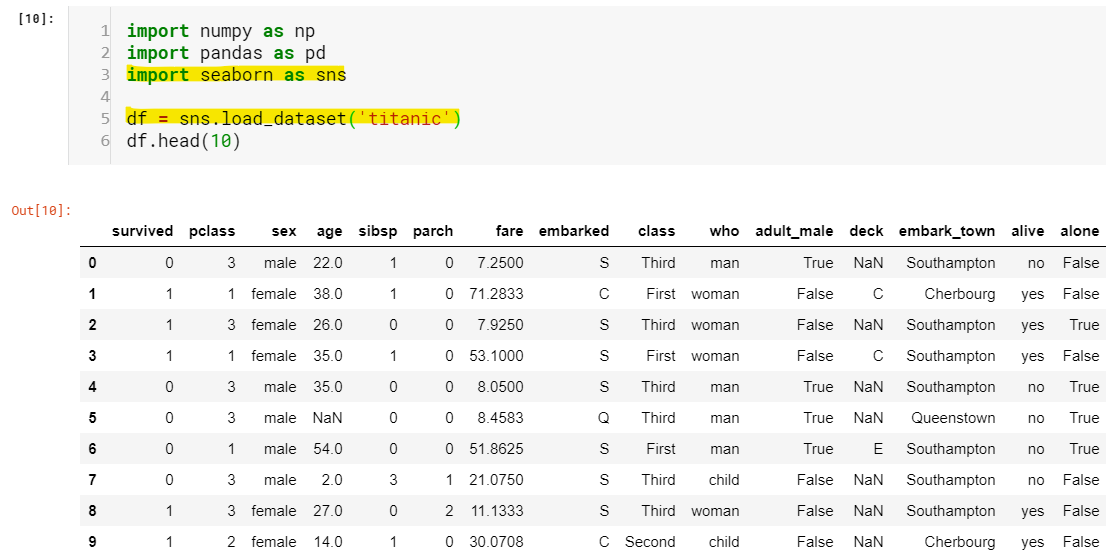# Unpacking Pandas for Data Science: Part 1Using the classic Titanic data to unleash the power of Pandas. If you are already familiar with NumPy, Pandas is just a package build on top of it. Pandas provide more flexibility than NumPy to work with data. While in NumPy we can only store values of single data type(dtype) Pandas has the flexibility to store values of multiple data type. Hence, we say Pandas is heterogeneous. We will unpack several more advantages of Pandas today.

If you are already familiar with NumPy, Pandas is just a package build on top of it. Pandas provide more flexibility than NumPy to work with data. While in NumPy we can only store values of single data type(dtype) Pandas has the flexibility to store values of multiple data type. Hence, we say Pandas is heterogeneous. We will unpack several more advantages of Pandas today.

Since we will be referring to NumPy in every section, I’m assuming you have knowledge of NumPy if not I will be dropping links to resources at the end of the article.

I’m considering the very popular _**_Titanic datset**_ to unpack the abilities of Pandas. You don’t have to worry because I will still be introducing the concepts of Pandas step-by-step keeping in mind you are a newbie to Pandas package._

Let’s just quickly import Pandas, Numpy, and load the Titanic dataset.Figure-0

I know, a lot to digest at once but we will break it down at the course of time. For now, don’t worry about line 3 and line 5(highlighted). Just understand that the seaborn package has the dataset in it and we loaded it, that’s all. You might have already figured out that ‘df’ holds our entire dataset but wait, what is the data type of 'df’? and what on Earth is ‘df.head(10)’. This brings us to our first topic Pandas objects: Series and DataFrame.

## Data Analysis | Data Analysis Projects | Data Science Projects | Exploratory Data Analysis | Pandas

In this tutorial, you will know about the TED TALKS DATA ANALYSIS project from scratch.

## Introduction to Data Analysis in Python | Python Pandas | Series | DataFrames | CSV | Plotting |

Introduction to Data Analysis in Python | Python Pandas | Series | DataFrames | CSV | Plotting | This course is aimed at the intermediate Python developer who wants to learn how to do useful data analysis tasks in Python. It will focus on the Python package pandas and matplotlib but will also include NumPy to some degree.

## Master Pandas’ Groupby for Efficient Data Summarizing And Analysis

Learn to group the data and summarize in several different ways, to use aggregate functions, data transformation, filter, map.

## Python for Data Science | Data Science With Python | Python Data Science Tutorial

🔥Intellipaat Python for Data Science Course: https://intellipaat.com/python-for-data-science-training/In this python for data science video you will learn e...

## An introduction to exploratory data analysis in python

Many a time, I have seen beginners in data science skip exploratory data analysis (EDA) and jump straight into building a hypothesis function or model. In my opinion, this should not be the case.